Courses

# JEE(MAIN) Physics Mock Test - 5

## 30 Questions MCQ Test JEE Main Mock Test Series 2020 & Previous Year Papers | JEE(MAIN) Physics Mock Test - 5

Description
This mock test of JEE(MAIN) Physics Mock Test - 5 for JEE helps you for every JEE entrance exam. This contains 30 Multiple Choice Questions for JEE JEE(MAIN) Physics Mock Test - 5 (mcq) to study with solutions a complete question bank. The solved questions answers in this JEE(MAIN) Physics Mock Test - 5 quiz give you a good mix of easy questions and tough questions. JEE students definitely take this JEE(MAIN) Physics Mock Test - 5 exercise for a better result in the exam. You can find other JEE(MAIN) Physics Mock Test - 5 extra questions, long questions & short questions for JEE on EduRev as well by searching above.
QUESTION: 1

### Emission of electrons in the photoelectric effect is possible, if

Solution: The wavelength should be low because lower the wavelength higher the frequency and for photo electric effect to occur we need a threshold frequency.
QUESTION: 2

Solution:
QUESTION: 3

### The acceleration due to gravity at a height 1/10th of the radius of the earth above the earth's surface is 8.2 m-s⁻1 Its value at a point at the same distance below the surface of the earth is

Solution:
QUESTION: 4
According to Hooke's law of elasticity, if stress is increased, the ratio of stress to strain
Solution:
QUESTION: 5
A 5 $\mu$F capacitor is fully charged across a 12V battery. It is then disconnected from the battery and connected to an uncharged capacitor. If the voltage across the capacitor becomes 3V, the capacitance of the uncharged capacitor is
Solution:
C1 * V1 = Q1. [Eq. 1]
Where, C1 = 5uF, V1= 12V.
This means capacitor 1 is able to gather up to 60uC of charge; before the circuit reaches voltage equilibrium and no more current flow is possible.
Now we transfer this bank of charge to another circuit with an uncharged capacitor, C2, which absorbs enough charge and leaves the circuit in equilibrium at 3V.
i.e. V2 = 3V
At 3V C1 can retain a charge of 15uC [using Eq. 1]. This means C2 most possess the remaining 45uC[Q2] of charge; at 3V[V2].
C2 = Q2/V2
C2= 45uC/3V
C2= 15uF
QUESTION: 6
If the error in the measurment of radius of a sphere is 2% , then the error in the determination of volume of the sphere will be
Solution:
QUESTION: 7

The volume of a gas will be double of what it is at 0oC (pressure remaining constant) at

Solution:
QUESTION: 8

An L.C. circuit contains 10 mH inductor and a 25 μ F capacitor. The resistance of the circuit is negligible. The energy stored in the circuit is completely magnetic at time (in milli seconds) being measured from the instant when the circuit is closed

Solution:

When R is negigible,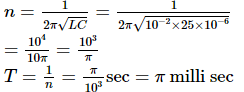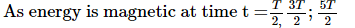Therefore, t = 1.57, 4.71, 7.85 ... milli sec

QUESTION: 9

The electric potential at any point (x,y,z) in a space is given by: V=4x2 volt. The electric field at (1,0,2) m in space is

Solution: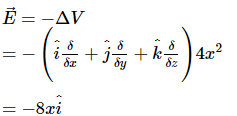Magnitude of electric field at the point (1m, 0, 2m) = − 8 V ∕ m

QUESTION: 10

The horizontal range of a projectile is R, when the angle of projection is 30o. The value of another angle of projection, for the same range, is

Solution:
QUESTION: 11
The first atomic reactor was constructed by
Solution:
QUESTION: 12
In the following question, a Statement of Assertion (A) is given followed by a corresponding Reason (R) just below it. Read the Statements carefully and mark the correct answer-
Assertion(A): A changing magnetic field acts as a source of electric field known as induced electric field.
Reason(R): Induced electric field can be produced by any static charge distribution.
Solution: A charged particle moving without acceleration produces an electric well as a magnetic field. It produces an electric field because it's a charge particle. But when it is at rest, it doesn't produce a magnetic field.So, when there will be no any magnetic field then not produce induced E.F.
QUESTION: 13
In the following question, a Statement of Assertion (A) is given followed by a corresponding Reason (R) just below it. Read the Statements carefully and mark the correct answer-
Assertion(A): Torque is an axial vector and directed along the axis of rotation.
Reason(R): Torque is equal to vector product of force to the position vector.
Solution:
QUESTION: 14
The astronomical telescope consists of objective and eye-piece. The focal length of the objective is
Solution:
QUESTION: 15
Frequency range of audible sound is
Solution:
QUESTION: 16
A stretched sonometer wire is in unison with a tuning fork of frequency f'. When the length of wire is increased by 2%, the number of beats produced per second is 5. The frequency of fork is
Solution:
QUESTION: 17
Bright colours exihibited by spider's web exposed to sun light are due to
Solution:
QUESTION: 18

If a ball of 80 kg mass hits an ice cube and temperature of ball is 100oC, how much ice becomes water? Specific heat of ball is 0.2 cal/gram.

Solution:
QUESTION: 19
A bullet of mass 50 gram is fired from a 5 kg gun with a velocity of 1 km/sec. The speed of recoil of the gun is
Solution:
QUESTION: 20

Two uncharged identical capacitors A and B, each of capacitance C, and an inductor of inductance L are arranged as shown in the adjacent figure. At t = 0, the switch S1 is closed while switch S2 remains open. At time t = t0=√LCπ/2, switch S2 is closed while switch S1 is opened.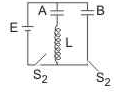Q. The charge on capacitor A after time t0 is

Solution:
QUESTION: 21

Two uncharged identical capacitors A and B, each of capacitance C, and an inductor of inductance L are arranged as shown in the adjacent figure. At t = 0, the switch S1 is closed while switch S2 remains open. At time t = t0=√LCπ/2, switch S2 is closed while switch S1 is opened.Q. The current flowing through the inductor at t = t0 is

Solution:
QUESTION: 22

Two uncharged identical capacitors A and B, each of capacitance C, and an inductor of inductance L are arranged as shown in the adjacent figure. At t = 0, the switch S1 is closed while switch S2 remains open. At time t = t0=√LCπ/2, switch S2 is closed while switch S1 is opened.Q.  After switch S2 is closed and S1 is opened, the maximum value of current through the inductor is

Solution:
QUESTION: 23

A small spherical monoatomic ideal gas bubble (γ=5/3) is trapped inside a liquid of density ρl as shown in figure. Assume that the bubble does not exchange any heat with the liquid. The bubble contains n moles of gas. The temperature of the gas when the bubble is at the bottom is T0, the height of the liquid is H and the atmospheric pressure is P0 (neglect surface Tension)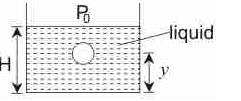Q. As the bubble moves upwards, besides the buouancy force the following forces are acting on it

Solution:
QUESTION: 24

A small spherical monoatomic ideal gas bubble (γ=5/3) is trapped inside a liquid of density ρl as shown in figure. Assume that the bubble does not exchange any heat with the liquid. The bubble contains n moles of gas. The temperature of the gas when the bubble is at the bottom is T0, the height of the liquid is H and the atmospheric pressure is P0 (neglect surface Tension)Q. When the gas bubble is at a height y from the bottom, its temperature is

Solution:
QUESTION: 25

A standing wave on a string is given by y = (4 cm) cos [ πx] sin [50 π t], where x is in metre and t is in seconds. The velocity of the string section at x = 1/3 m at t = 1/5 s, is

Solution:
QUESTION: 26

A conducting wheel having 24 spokes, out of which 12 are conducting and 12 are non-conducting, is rotating about its own axis with constant angular velocity ωo . A uniform magnetic field B0 is present in the region directed into the plane of paper as shown in the figure. If the radius of wheel is R, then the emf induced between the centre of wheel and its periphery is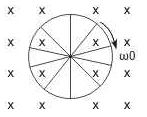Solution:
QUESTION: 27

The x - t graph of a particle undergoing simple harmonic motion is shown below. The acceleration of the particle at t = 4/3 s is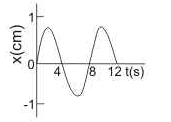Solution:
QUESTION: 28

An harmonic wave has been set up on a very long string which travels along the length of string. The wave has frequency of 50 Hz. amplitude 1 cm and wavelength 0.5 m. For the above described wave
Statement I : Time taken by the wave to travel a distance of 8 m along the length of string is 0.32 s.
Statement II : Time taken by a point on the string to travel a distance of 8 m, once the wave has reached at that point and sets it into motion, is 4s.

Solution:
QUESTION: 29

A mass M collides elastically with a stationary mass m. If M < m, then it is possible for M to bounce directly backwards. However, if M > m, then there is a maximum angle of deflection of M. Then angle is

Solution:
QUESTION: 30

A train of mass M is moving on a circular track of radius R with constant speed V. The length of the train is half of the perimeter of the track. The linear momentum of the train will be

Solution: